##### Calculus II For DummiesImproper integrals are useful for solving a variety of problems. A horizontally infinite improper integral contains either ∞ or –∞ (or both) as a limit of integration.

Evaluating an improper integral is a three-step process:

1. Express the improper integral as the limit of a proper integral.

2. Evaluate the integral by whatever method works.

3. Evaluate the limit.

A horizontal improper integral occurs when a definite integral has a limit of integration that’s either ∞ or –∞. This type of improper integral is easy to spot because infinity is right there in the integral itself. You can’t miss it.

For example, suppose that you want to evaluate the following improper integral: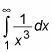Here’s how you do it, step by step:

1. Express the improper integral as the limit of a proper integral.

When the upper limit of integration is ∞, use this equation: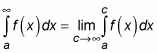So here’s what you do: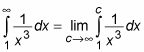2. Evaluate the integral: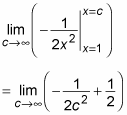3. Evaluate the limit: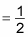Before moving on, reflect for one moment that the area under an infinitely long curve is actually finite. Ah, the magic and power of calculus!

Similarly, suppose that you want to evaluate the following: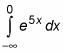Here’s how you do it:

1. Express the integral as the limit of a proper integral.

When the lower limit of integration is –∞, use this equation: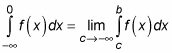So here’s what you write: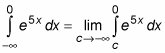2. Evaluate the integral: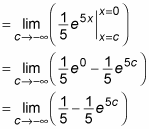3. Evaluate the limit — in this case, as c approaches –∞, the first term is unaffected and the second term approaches 0: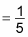Again, calculus tells you that, in this case, the area under an infinitely long curve is finite.

Of course, sometimes the area under an infinitely long curve is infinite. In these cases, the improper integral can’t be evaluated because the limit does not exist (DNE). Here’s a quick example that illustrates this situation: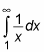It may not be obvious that this improper integral represents an infinitely large area. After all, the value of the function approaches 0 as x increases. But watch how this evaluation plays out:

1. Express the improper integral as the limit of a proper integral: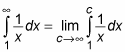2. Evaluate the integral: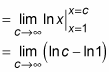At this point, you can see that the limit explodes to infinity, so it doesn’t exist. Therefore, the improper integral can’t be evaluated, because the area that it represents is infinite.